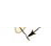Chapter 8, Problem 7P

Chapter
Section
Textbook Problem

A simple pendulum consists of a small object of mass 3.0 kg hanging at the end of a 2.0-m-long light string that is connected to a pivot point. (a) Calculate the magnitude of the torque (due to the force of gravity) about this pivot point when the string makes a 5.0° angle with the vertical. (b) Does the torque increase or decrease as the angle increases? Explain.Figure P8.6

(a)

To determine
The magnitude of the torque due to gravity about the pivot point.

Explanation

Given info: The length of the light string is 2.0m , mass of the small object is 3.0kg , and acceleration due to gravity is 9.8m/s2 .

The formula for the magnitude of the torque of the pendulum is,

τ=lmgsinθ

• l is length of the string.
• m is mass of the object.
• g is acceleration due to gravity.
• θ is the angle of the force.

Substitute 3.0kg for m , 9.8m/s2 for g , 2.0m for l , and 5

(b)

To determine
The torque increase or decrease as the increase of the angle.

Still sussing out bartleby?

Check out a sample textbook solution.

See a sample solution

The Solution to Your Study Problems

Bartleby provides explanations to thousands of textbook problems written by our experts, many with advanced degrees!

Get Started

Find more solutions based on key concepts